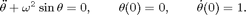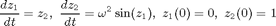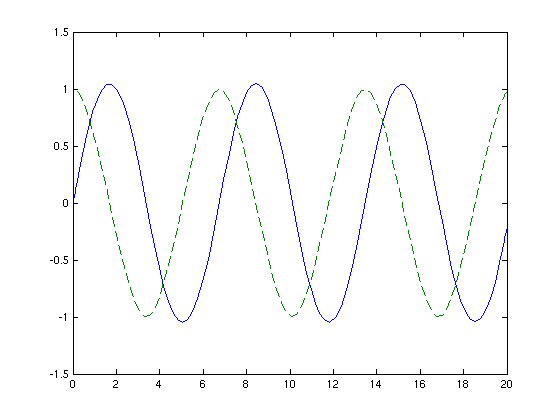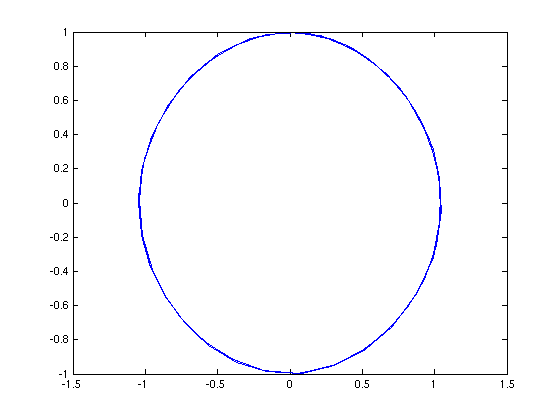# A nonlinear pendulum

## The differential equation:## The corresponding system of the first order differential equations:## Input initial conditions:

z0=[0,1];


## Define the interval on which solution is computed:

tspan =[0,20];


## Solve the system using ode45 procedure:

[t,z] = ode45('ode3',tspan,z0);


## Extract the positions and velocities:

x=z(:,1); v=z(:,2);


## Plots of the positions and velocities as functions of time:

Note: The dashed curve indicates velocities

plot(t,x,t,v,'--')## Plot of the phase portrait (velocity as the function of position):

figure(2)
plot(x,v)